Goseeko blog# What are Logic Gates?

The logic gates are the most basic circuits used in digital electronics. It is the device with two or more inputs and only one output. There are different types of gates used to obtain different logical expressions. The function of each logic gate is represented by Boolean expression.

## Types of Logic Gates

They can be classified as:

1. Basic gates
2. Universal gates
3. Special gates

## Basic Logic gates

They are AND gates, OR gates and NOT gates.

AND gate: It is a digital circuit that consists of two or more inputs and a single output which is the logical AND of all those inputs. The symbol for AND gate is ‘.’. The circuit for OR gate is below.OR gate

It is a digital circuit which has two or more inputs and a single output which is the logical OR of all those inputs. The symbol for OR gate is ‘+’.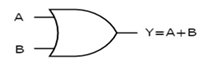NOT gate

It is a digital circuit that has one input and one output. Here the output is the logical inversion of input. Hence, it is also called an inverter.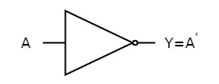## Universal Logic Gates

NAND & NOR gates are known as universal gates. We can implement any Boolean function by using NAND gates and NOR gates alone.

NAND gate: It is a digital circuit which has two or more inputs and single output and it is the inversion of logical AND gate. The image shows the symbol of NAND gate: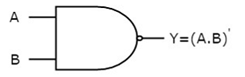NOR gate: It is a digital circuit that has two or more inputs and a single output which is the inversion of logical OR of all inputs. The image shows the symbol of NOR gate.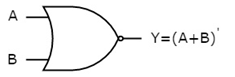## Special Gates

Ex-OR gate: It stands for Exclusive-OR gate. Its function varies when the inputs have an even number of ones. The output of Ex-OR gate is ‘1’, when only one of the two inputs is ‘1’. And it is zero, when both inputs are the same. The output can be known as odd function. The symbol for EX-OR gate is below.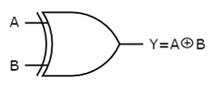Ex-NOR gate

It stands for Exclusive-NOR gate. Its function is the same as that of NOR gate except when the inputs have an even number of ones. Hence the output of Ex-NOR gate is ‘1’, when both inputs are same and 0, when both the inputs are different. The symbol is for Ex-NOR gate is below.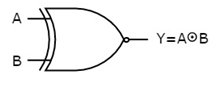Interested in learning about similar topics? Here are a few hand-picked blogs for you!